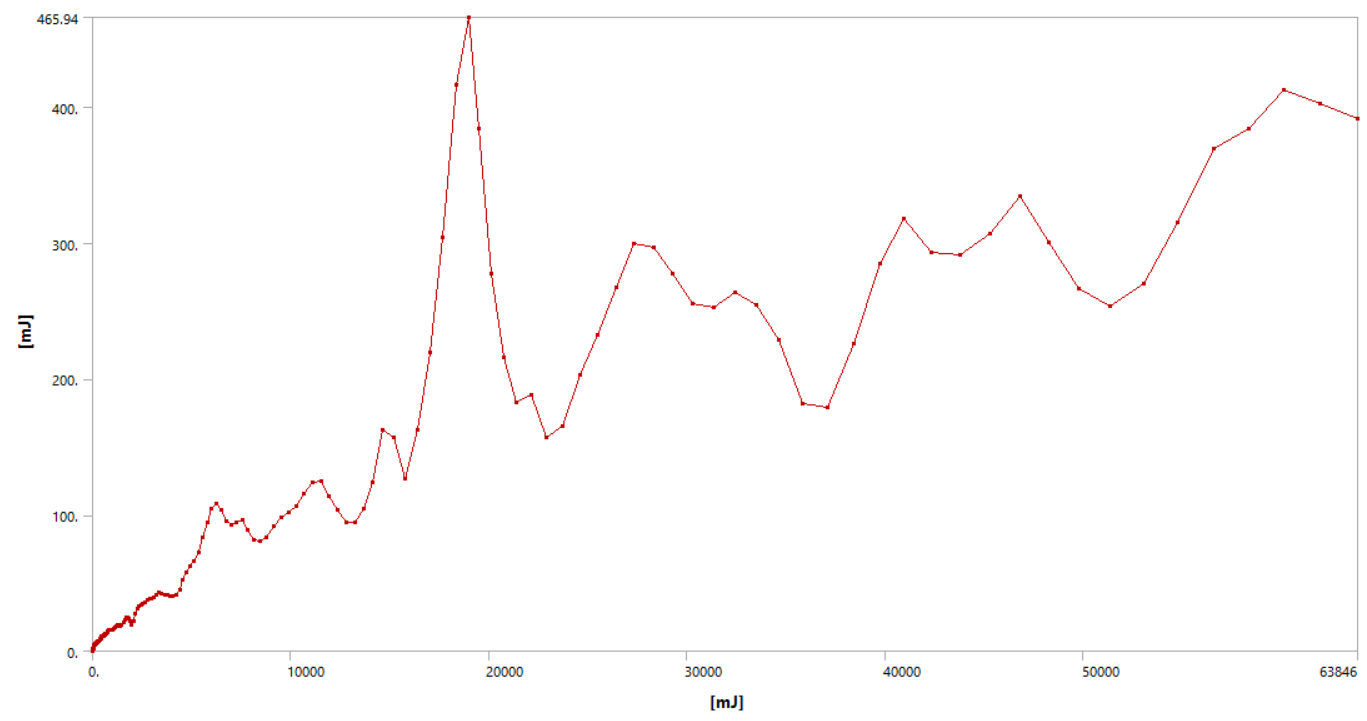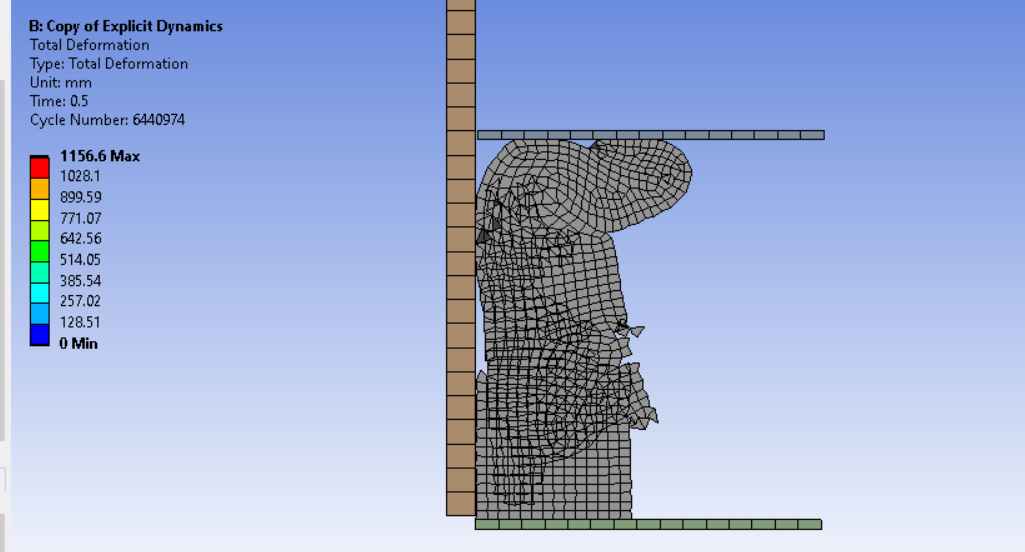Mahdi_171
Subscriber
Dear Peter,nThank you so much. It would have taken me much more time to reach this point. I spent some time reading about the topic and I found this equation.nThe reference says that the loading velocity should be less than 1% of the material wave velocity (estimated by the previous equation) to minimize the inertia effect. I changed the elastomer to a type with higher Young's modulus to see what happens and I got this plot for the kinetic vs total energy.nI don't know if I can consider this simulation as quasi-static so I tried a slower one with constant velocity and the energy plot was much better with almost zero kinetic energy. However, the elements crushed at one point although the simulation was completed.nCould this be a result of high-frequency that you mentioned before?.I want to ask another question if possible. I want to view the 3d deformed model so I tried cyclic symmetry but the option was hidden and the help section doesn't explain anything about that.n